## Centroid (Triangle)

The centroid (Center of Mass) of the Vertices of a Triangle is the point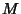(or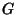) of intersection of the Triangle's three Medians, also called the Median Point (Johnson 1929, p. 249). The centroid is always in the interior of the Triangle, and has Trilinear Coordinates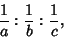(1)

or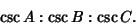(2)

If the sides of a Triangle are divided so that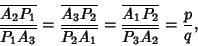(3)

the centroid of the Triangle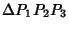is(Johnson 1929, p. 250).

Pick an interior point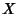. The Triangles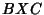,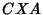, and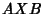have equal areas Iffcorresponds to the centroid. The centroid is located one third of the way from each Vertex to the Midpoint of the opposite side. Each median divides the triangle into two equal areas; all the medians together divide it into six equal parts, and the lines from the Median Point to the Vertices divide the whole into three equivalent Triangles. In general, for any line in the plane of a Triangle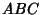,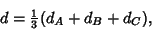(4)

where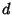,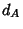,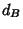, and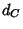are the distances from the centroid and Vertices to the line. A Triangle will balance at the centroid, and along any line passing through the centroid. The Trilinear Polar of the centroid is called the Lemoine Axis. The Perpendiculars from the centroid are proportional to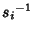,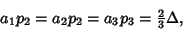(5)

where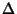is the Area of the Triangle. Let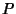be an arbitrary point, the Vertices be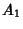,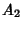, and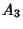, and the centroid. Then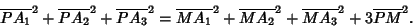(6)

If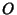is the Circumcenter of the triangle's centroid, then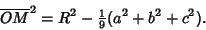(7)

The centroid lies on the Euler Line.

The centroid of the Perimeter of a Triangle is the triangle's Spieker Center (Johnson 1929, p. 249).

References

Carr, G. S. Formulas and Theorems in Pure Mathematics, 2nd ed. New York: Chelsea, p. 622, 1970.

Coxeter, H. S. M. and Greitzer, S. L. Geometry Revisited. Washington, DC: Math. Assoc. Amer., p. 7, 1967.

Dixon, R. Mathographics. New York: Dover, pp. 55-57, 1991.

Johnson, R. A. Modern Geometry: An Elementary Treatise on the Geometry of the Triangle and the Circle. Boston, MA: Houghton Mifflin, pp. 173-176 and 249, 1929.

Kimberling, C. Central Points and Central Lines in the Plane of a Triangle.'' Math. Mag. 67, 163-187, 1994.

Kimberling, C. Centroid.'' http://cedar.evansville.edu/~ck6/tcenters/class/centroid.html.